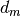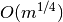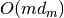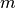Warning

This documents an unmaintained version of NetworkX. Please upgrade to a maintained version and see the current NetworkX documentation.

# random_degree_sequence_graph¶

random_degree_sequence_graph(sequence, seed=None, tries=10)[source]

Return a simple random graph with the given degree sequence.

If the maximum degreein the sequence isthen the algorithm produces almost uniform random graphs intime whereis the number of edges.

Parameters: sequence (list of integers) – Sequence of degrees seed (hashable object, optional) – Seed for random number generator tries (int, optional) – Maximum number of tries to create a graph G – A graph with the specified degree sequence. Nodes are labeled starting at 0 with an index corresponding to the position in the sequence. Graph NetworkXUnfeasible – If the degree sequence is not graphical. NetworkXError – If a graph is not produced in specified number of tries

See also

is_valid_degree_sequence(), configuration_model()

Notes

The generator algorithm  is not guaranteed to produce a graph.

References

  Moshen Bayati, Jeong Han Kim, and Amin Saberi, A sequential algorithm for generating random graphs. Algorithmica, Volume 58, Number 4, 860-910, DOI: 10.1007/s00453-009-9340-1

Examples

>>> sequence = [1, 2, 2, 3]
>>> G = nx.random_degree_sequence_graph(sequence)
>>> sorted(G.degree().values())
[1, 2, 2, 3]